# Spring Test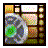## Watch the video tutorial on using a spring!

In this test we will build a standard mass-spring-damper system to verify the functionality of the spring body part. This system is depicted in figure 1. It has a block mass connected to a non-moving object with a spring and a dashpot. The mathematical description for this system is shown in equation 1.(1)

K is the stiffness of the spring. Delta x is the displacement of the spring from its resting length. B is the damping coefficient, and v is the velocity of the mass. So the spring exerts a force that is proportional to the amount it is stretched or compressed. The damper produces forces proportional to how fast the mass is moving. So the faster the block tries to move, the harder the dashpot pushes back on it to resist that movement.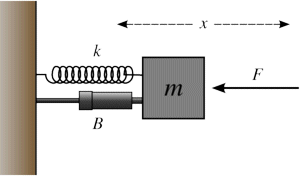Figure 1. Standard mass-spring-damper system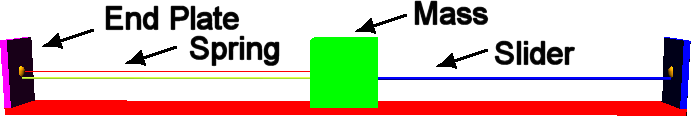Figure 2. Virtual test rig for the spring-damper system.

We will be using the test rig shown in figure 2. It has a base with endplates on either side. A block mass is connected to the base using a prismatic joint. This joint only allows the mass to slide back and forth along the base. It prevents it from moving in any other direction. Attachment sites are located on the left side and the center of the mass, and a spring-damper is connected to each of these attachment sites. System of this type produce oscillatory movements if disturbed. So if you were to pull the block so that the spring is no longer at its natural length it will spring back and oscillate around its resting length. If there is no damping then you have just a spring-mass system which has a natural frequency at which it oscillates. The equation to calculate the natural frequency is given in equation 2.(2)

However, if the damping coefficient is non-zero then the system will not actually oscillate at its natural frequency. Instead it will operate at a lower frequency because the damper will tend to slow it down. Equation 3 shows how to calculate the new frequency of the system taking into account the damping.(3)

If you solve differential equation 1 you will get equation 4 that describes the position of the block over time if it starts out moved from the springs natural length L0 by the amplitude A(4)

Similarly you can calculate the velocity and acceleration of the block by taking the first and second derivative of equation 4. These are shown in equations 5 and 6.(5)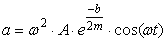(6)

Finally, you can also calculate the potential energy that is stored in a spring using equation 7.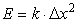(7)

All tests will use K = -10 N/m, m = 1 kg, A = 1 m, L0 = 4 m, and a time step of t = 1 ms. We can use this to calculate the natural frequency in equation 8. The maximum potential energy is E = 1/2*10*1^2 = 5 Joules. The maximum tension developed is F = K*A = 10*1 = 10 Newtons.(8)

### Un-damped spring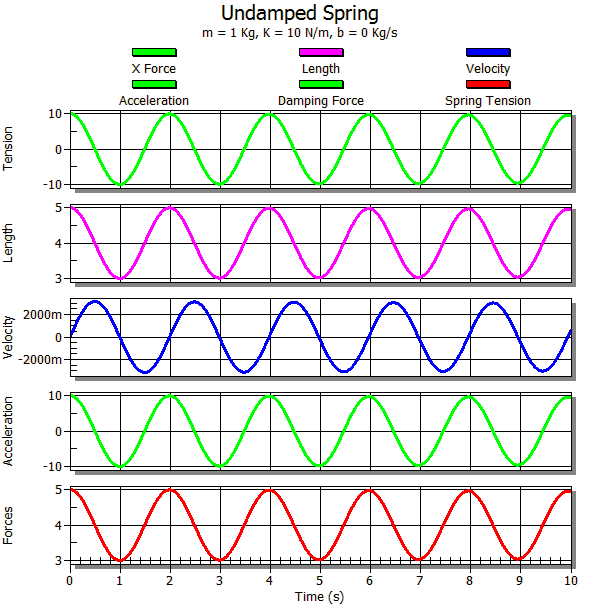Figure 3. Output data from the un-damped spring-mass system.

We will begin our tests by looking at the un-damped case where b=0. In this case the system should oscillate at the natural frequency. Figure 3 shows the output from this test. Notice that the oscillations occur around the 4 meter mark and that they maintain a consistent amplitude over time because there is no damping or friction losses to slow the oscillations. The maximum tension oscillates between -10 and 10 Newton's as expected. The peak velocity matches our predicted value of 3.16 m/s. The period of the oscillation is calculated using T = 2*PI/w = 1.988 seconds. The period of the simulated mass crosses the zero mark between 1.986 and 1.987 seconds. The peak acceleration is 10.5 m/s^2, which is slightly different than the calculated value of 9.986. The reason for this is that we are approximating the instantaneous velocity by using the successive position values divided by the time step. Then acceleration is done the same way using successive velocity values. These approximations lead to small inaccuracies. Finally, the peak potential energy matches the calculated value of 5 Joules.

### Under-damped spring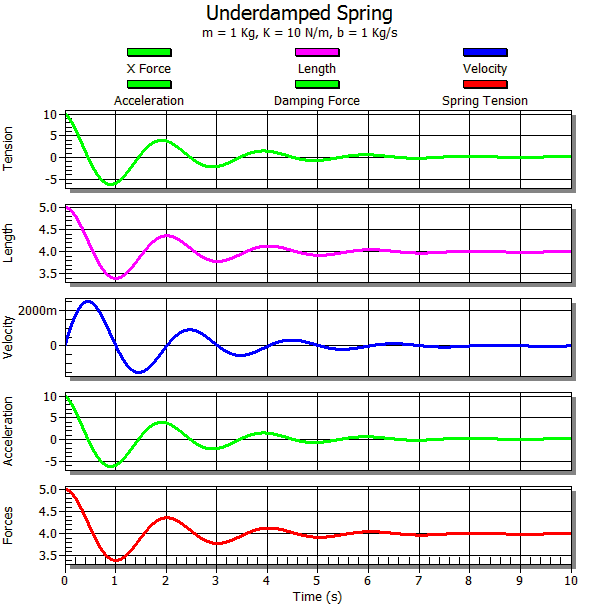Figure 4. Output data from the under-damped spring-mass system.

An under-damped spring is one where the damping is not strong enough to completely eliminate oscillations. We will set b = 1 Kg/s for this test. This gives us a new oscillation frequency w = 3.12 rad/s, and a new period of 2.014 seconds. Figure 4 shows the data output from AnimatLab. Unlike the previous simulation the oscillations quickly die out so that the mass comes to rest at the natural length of the spring. Figure 5 shows the calculated position and velocity curve using equations 4 and 5 above. Figure 6 shows the error percentage between the simulated and calculated position and velocity values normalized to the maximum values. The maximum value that the position reaches is 5 meters, so if the error was 5 meters then the error percentage in that case would be 100%. The simulation has a maximum positional error of 0.066% of the maximum position, and a maximum velocity error of 0.123% of the maximum velocity. You can reduce the error rates by making the time step you are using smaller.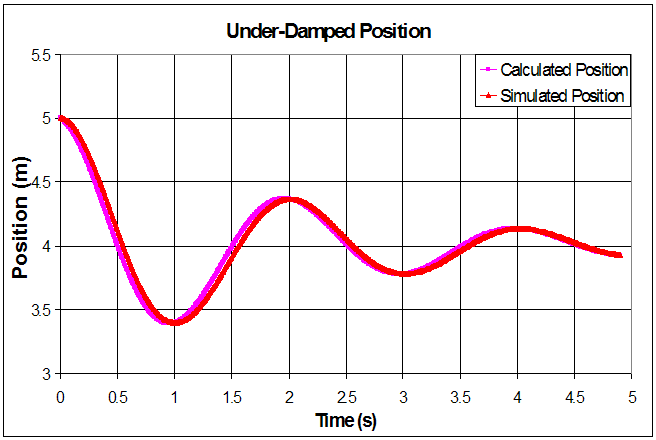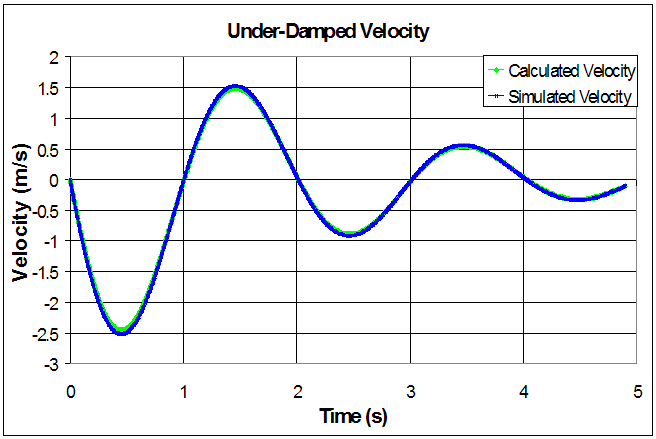Figure 5. Calculated position and velocity curves for the under-damped case.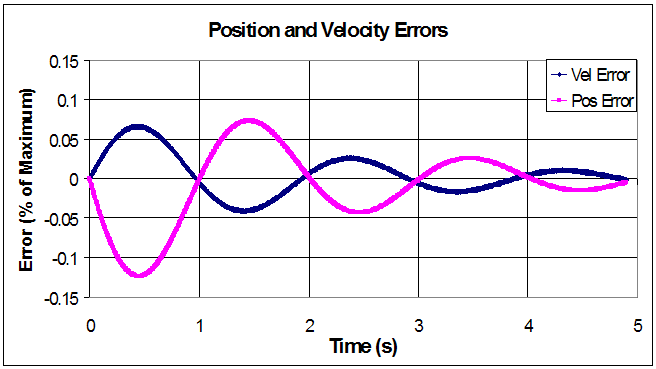Figure 6. Error between the simulated and calculated position and velocity of the under-damped spring system.

### Critically-damped spring

A critically damped system is one where the damping ratio is just high enough to make the eigen values of the differential equation become real. This means that the spring-mass system no longer oscillates at all. Instead the position decays exponentially to the equilibrium position. We can find the equations for the motion of the mass and its velocity using the equations below. One important point to notice here is that the roots of the characteristic equation are repeated and real. This happens because the damping coefficient is just right so that the square root portion of the quadratic equation is zero. The damping for this case is can be calculated using the equation b=2mw0, resulting in b=6.32 Kg/s.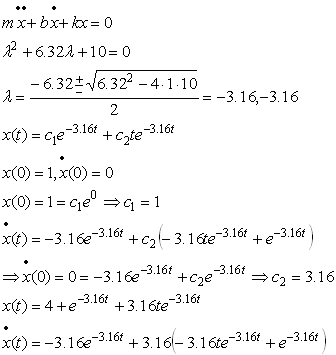Figure 7. Output data from the critically-damped spring-mass system.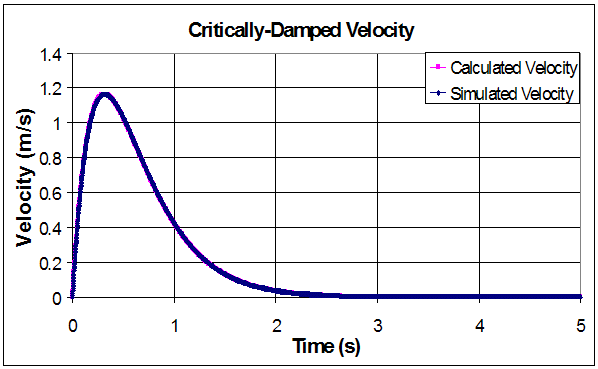Figure 8. Calculated position and velocity curves for the critically-damped case.Figure 9. Error between the simulated and calculated position and velocity of the critically-damped spring system.

The output of the simulated system matches well with the output from the derived equations. The simulation has a maximum positional error of 0.0077% of the maximum position, and a maximum velocity error of 0.077% of the maximum velocity.

### Over-damped spring

An overdamped system has a damping coefficient that is higher than the critical damping value. When the system is critically damped it does not oscillate and the position drops to the rest state as quickly as possible. If you increase the damping beyond this point then you will slow the drop to the rest position even more. We derive the equation of motion of the block and the equation for its velocity below. The damping for this case is 10 Kg/s.Figure 10. Output data from the over-damped spring-mass system.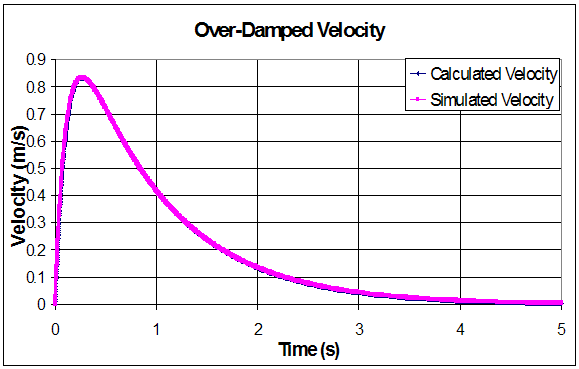Figure 11. Calculated position and velocity curves for the over-damped case.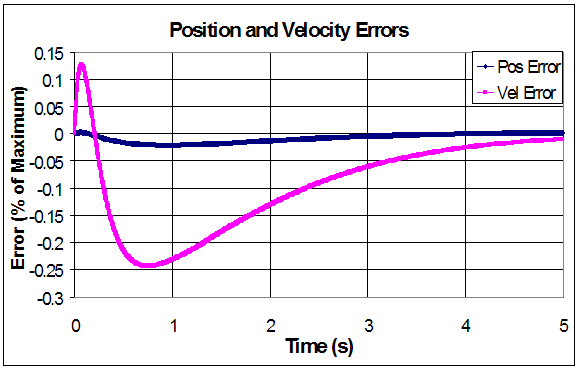Figure 12. Error between the simulated and calculated position and velocity of the over-damped spring system.

The output of the simulated system matches well with the output from the derived equations. The simulation has a positional error of 0.0209% of the maximum position, and a velocity error of 0.0243% of the maximum velocity.